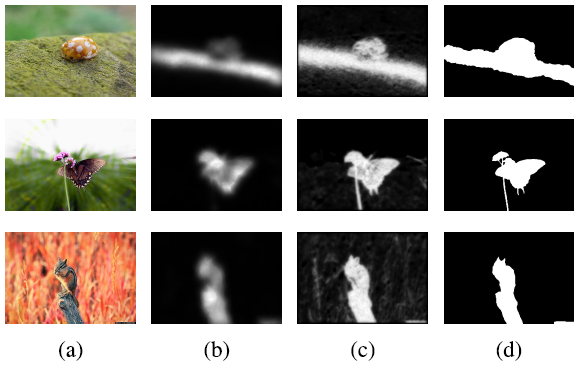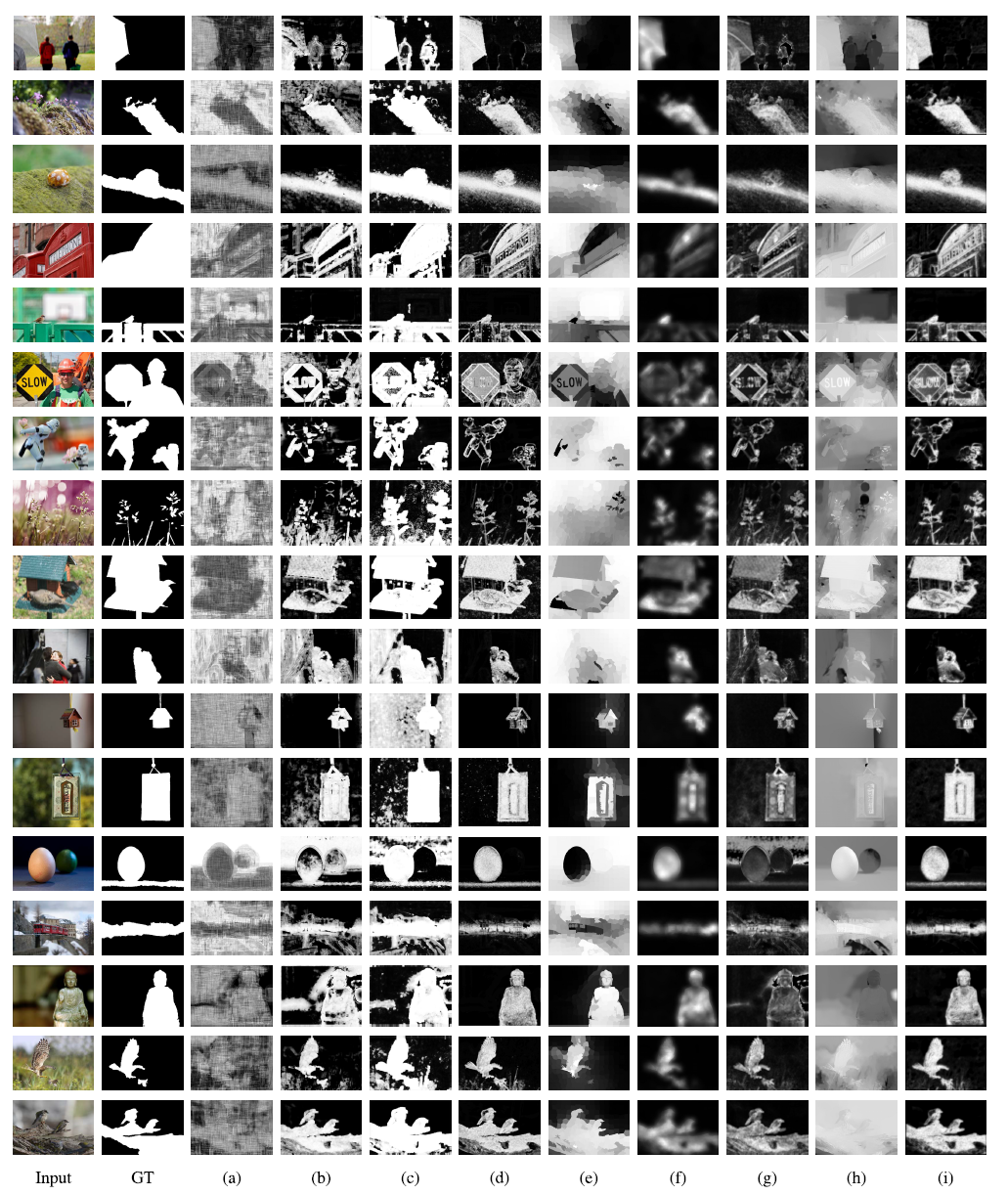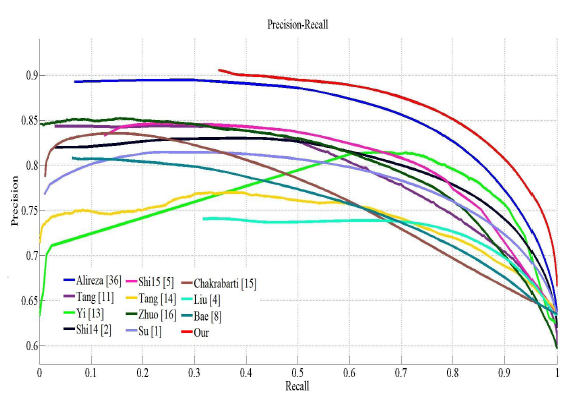# Review — Zeng TIP’19: A Local Metric for Defocus Blur Detection Based on CNN Feature Learning (Blur Detection)

## CNN + PCA for Feature Learning, Iterative Refinement Using Tanh(a) Input, (b) SOTA , (c) Proposed, (d) Ground Truth

In this story, A Local Metric for Defocus Blur Detection Based on CNN Feature Learning, Zeng TIP’19, Hunan University, and National Engineering Laboratory for Robot Visual Perception and Control Technology, is reviewed. In this paper:

• The ConvNets automatically learn the most locally relevant features.
• By extracting convolution kernels from the trained neural network structures and processing it with principal component analysis (PCA), the local sharpness metric is automatically obtained by reshaping the principal component vector.
• An effective iterative updating mechanism is proposed to refine the defocus blur detection result from coarse to fine by hyperbolic tangent function.

This is a paper in 2019 TIP where TIP has a high impact factor of 9.34. (Sik-Ho Tsang @ Medium)

# Outline

1. Overall Approach
2. Data Preparation
3. Proposed CNN: Network Architecture
4. Principal Component Analysis (PCA) for the Convolutional Kernels
5. Iterative Updating Mechanism Using Tanh
6. Experimental Results

# 1. Overall Approach

• Unlike SOTA CNN approaches, authors do not intend to construct a convolutional neural networks architecture for defocus estimation and classification.

The goal is only to use the ConvNets to automatically learn the most locally relevant features in unblurred or blurred region.

• Also unlike SOTA hand-crafted feature approaches, putting a lot of effort into designing the blur detection metrics. authors have automatically obtain the local metric extracted from the convolutional kernels of the trained ConvNets, which no need to know any prior information of the defocus image.

And then a novel iterative mechanism is proposed to refined the detection results.

# 2. Data Preparation

• The ConvNets trained database is constructed by extracting equal-sized patches around desired points of interest in the blurry or non-blurry region.
• For the local patches extraction, super-pixels  are extracted by clustering the homogeneous pixels.
• Afterward, a patch is extracted by centering a s×s window at the centroid of each super-pixel.
• According to the accompanying hand-segmented ground-truth images, the category of patches are determined by applying a threshold to the ratio of blurred or sharp regions that occupy the entire patch area.

Hence, there are two datasets have been constructed: the blur patch dataset and sharpness patch dataset.

# 3. Proposed CNN: Network Architecture

• The ConvNets architecture used for feature learning consists of alternating multi-convolution and sub-sampling layers, which are similar to VGG.
• The last layer of the ConvNets is the fully connected layer.
• The output is a logistic regression layer that provides a distribution over the classes. The output layer includes just two classes, i.e., for the blurry and sharpness feature learning.
• A batch normalization algorithm is used to normalize the input data.
• CReLu is used.
• The cross-entropy loss function is used.

Hence, the ConvNets can automatically learn the image feature representations.

# 4. Principal Component Analysis (PCA) for the Convolutional Kernels

• The convolutional kernels are extracted from the trained ConvNets architecture and then its principal components are analyzed.
• The principal feature, in other words, the local sharpness metric map or blur detection detector, is acquired.
• Each convolution kernel is reshaped into a column, all the convolution kernels are concatenated into a matrix, and then PCA is used to extract the principal components of the matrix.
• Finally, the principal component vector that has the maximum explained
• variance ratio, is reshaped.

For example, the convolutional kernel scale is 7×7 and there are N convolution kernels for the trained ConvNets architecture.

So, the convolutional kernel matrix size is 49×N, and the matrix size for the PCA result is 49×K, where K is the dimensionality of PCA after dimension reduction.

• K = 2, which mean there are two local metric maps have been reshaped: K1 and K2.
• where I denote the defocus image, L1 and L denote the detection result for the local metric.

# 5. Iterative Updating Mechanism Using Tanh

• The tanh function is a non-linear function and increases in a strictly monotonic curve whose output values are limited within the range of [−1,1]. When the input value is larger than zero, the output value is always less than the input value.
• The unblurred regions have higher intensities than the blurred ones and pixels value is within the range [0, 1].
• The aim of the refined mechanism is to enhance the response of the unblurred region and reduce the response of the blurred region.

If the neighborhood means is less than the global threshold, the output is zero, which always occurs in the blurred region.

If the neighborhood means is greater than or equal to the global threshold, the output is calculated by the tanh function, and this phenomenon always appears in the non-blurred region.

• The iterative refined results BN1 , BN2 , BN3 are combined to get the final results:
• where the iterative numbers N = 100. During the iterative refined of detection results, denote N1 = 10, N2 = 50, N3 = 100 and α1 = 0.3, α2 = 0.2, α3 = 0.5.
• (There are many thresholds in the paper. If interested, please feel free to read the paper.)

# 6. Experimental ResultsResults achieved by different blur detection methods (a)-(h): SOTA, (i) Proposed Approach
• Shi’s dataset is used.
• The above figure shows examples of detection results by the sharpness maps for each algorithm.The comparison of precision-recall curves for different methods
• In this experiment, the binarized results for the blur detection maps are segmented by applying the adaptive threshold.
• The precision within almost the entire recall range [0, 1], which demonstrates the superiority of our proposed method.
• The resolution of the test image is 640×457 pixels.
• The proposed approach has a very short running time.
• (There are some experiments on choosing threshold values in the paper. If interested, please feel free to read the paper.)

## Reference

[2019 TIP] [Zeng TIP’19]
A Local Metric for Defocus Blur Detection Based on CNN Feature Learning

## Blur Detection / Defocus Map Estimation

2017 [Park CVPR’17 / DHCF / DHDE] 2018 [Purohit ICIP’18] [BDNet] [DBM] [BTBNet] 2019 [Khajuria ICIIP’19] [Zeng TIP’19] 2020 [BTBCRL (BTBNet + CRLNet)]

--

--